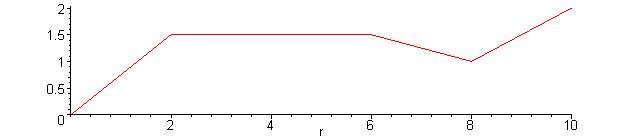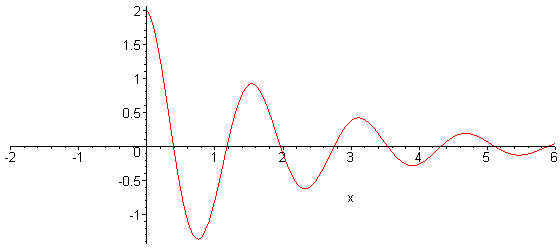# Introduction

One of the causes of polynomial wiggle is using too many points in the interpolating polynomial. We introduce the most basic piecewise interpolating function where a different interpolating polynomial is used on each interval. Piecewise linear interpolations fall into a class of functions called splines. Topics 5.9 and 5.10 go into further depth with two other types of splines.

# Theory

The interpolating polynomials which have been seen to this point have been defined on for all the n points (x1, y1), (x2, y2), ..., (xn, yn). An alternative approach is to define a different interpolating polynomial on each sub-interval under the assumption that the x values are given in order.

The simplest means is to take each pair of adjacent points and find an interpolating polynomial between the points which using Newton polynomials is

This can be expanded to reduce the number of required operations by reducing it to a form ax + b which can be computed immediately. The reader may note that if the value x = xk + 1 is substituted into the above equation that the value is yk + 1.

A significant issue with piecewise linear interpolation is that the interpolant is not differentiable or smooth. A non-differentiable function can introduce new issues in a system almost as easily as a non-continuous function.

# HOWTO

Given a set of n points (x1, y1), (x2, y2), ... (xn, yn) where x1 < x2 < ··· < xn, a piecewise linear function is defined for a point x such that xkxxk + 1 by

# Error Analysis

The error for a piecewise linear interpolant is the error on each sub-interval

where ξ(x) is a point in the interval and may be different for each x.

A little algebra shows that the maximum of the polynomial component (x − xk)(x − xk + 1) must be less in absolute value than ¼(xk + 1 − xk)2; consequently, we find the less strict bound

where sup finds the least upper bound for the its arguement.

# Examples

1. Find the peicewise linear interpolating polynomial for the points (0, 0), (2, 1.5), (6, 1.5), (8, 1), (10, 2).

In Matlab, we can perform one step of the divided difference formula:

```>> x = [0 2 6 8 10]';
>> y = [0 1.5 1.5 1 2]';
>> a = (y(1:4) - y(2:5)) ./ (x(1:4) - x(2:5))
a =
0.75000
0.00000
-0.25000
0.50000
```

After simplification, this yeilds

which has the plotFigure 1. The piecewise linear interpolation of the points (0, 0), (2, 1.5), (6, 1.5), (8, 1), (10, 2).

# Applications to Engineering

In general, linear piecewise interpolation is almost always inappropriate for engineering unless:

• The function is almost linear,
• A large error is acceptable.

Examples where linear interpolation may be used include computer graphics where another subroutine has determined how many points are required to compensate for the subsequent linear interpolation. The section on Maple demonstrates how linear interpolation may be used in graphics.

# Matlab

The following code finds the

```% return two (n-1)-dimensional vectors so that a(k)*x + b(k) is the
% linear interpolating polynomial between points x(k) and x(k + 1)
function [a, b] = piecewise( x, y )
n = length( x );
a = (y( 2:n ) - y( 1:(n - 1) )) ./ (x( 2:n ) - x( 1:(n - 1) ));
b = (y( 1:(n - 1) ).*x( 2:n ) - y( 2:n ).*x( 1:(n - 1) )) ./ (x( 2:n ) - x( 1:(n - 1) ));
end
```

# Maple

Figure 1 shows a Maple plot of the function sin(4x)e-x/2u(x).

```> plot( 2*Heaviside(x)*exp(-x/2)*cos(4*x), x = -2..6 );
```Figure 2. A Maple plot.

The plot is generated by linearly interpolating a number of points where first fifty points are sampled and then additional subsamples where the points do not appear to be sufficiently smooth according to certain heuristics. The interpolated points are shown in Figure 3.

```> plot( 2*Heaviside(x)*exp(-x/2)*cos(4*x), x = -2..6, style = point, symbol = circle );
```Figure 3. The points interpolated in making the maple plot.

It is possible to turn off the adaptive subsampling to reveal the first fifty samples as shown in Figure 4.

```> plot( 2*Heaviside(x)*exp(-x/2)*cos(4*x), x = -2..6, adaptive = false );
```Figure 4. The initial 50 points without adaptive subsampling.

Interpolating an insufficient number of points will result in a less pleasing result shown in Figure 5.

```> plot( 2*Heaviside(x)*exp(-x/2)*cos(4*x), x = -2..6, style = point, symbol = circle, adaptive = false );
```Figure 5. The linear interpolation of 50 points not using subsampling.

Maple also allows the user to state the number of initially sampled points and require the plotting function to first determine discontinuties.

```> plot( 2*Heaviside(x)*exp(-x/2)*cos(4*x), x = -2..6, symbol = circle, style = point, numpoints = 1000, discont = true );
```Figure 6. Starting with a larger number of initial points and allowing Maple to determine discontinuities.